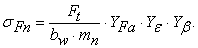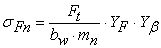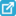﻿ Stress Analysis of Eccentric Gear with Asymmetrical Profile of Teeth

### Stress Analysis of Eccentric Gear with Asymmetrical Profile of Teeth

Silvia Medvecká-Beňová, František Trebuňa, Peter Frankovský

American Journal of Mechanical Engineering

## Stress Analysis of Eccentric Gear with Asymmetrical Profile of Teeth

Silvia Medvecká-Beňová1,, František Trebuňa2, Peter Frankovský3

1Department of Construction, Automotive and Transport Engineering, Faculty of Mechanical Engineering, Technical University of Košice, Košice, Slovakia

2Department of Applied Mechanics and Mechanical Engineering, Faculty of Mechanical Engineering, Technical University of Košice, Košice, Slovakia

3Department of Mechatronics, Faculty of Mechanical Engineering, Technical University of Košice, Košice, Slovakia

### Abstract

The bending stress of gearing defined by calculation and the actual values of teeth stress may be vary significantly. The stress calculated in a standard way according to available formulas is approximate because some impacts cannot be determined with sufficient accuracy. Recently, at ever faster evolving computer technology, the available literature, we can meet with modern numerical methods, such as finite element method (FEM). The article is devoted to problems determining of the stress in a dangerous section of tooth foot using FEM. The problem is solved for elliptical, eccentric gear with asymmetrical profile of tooth.

• Silvia Medvecká-Beňová, František Trebuňa, Peter Frankovský. Stress Analysis of Eccentric Gear with Asymmetrical Profile of Teeth. American Journal of Mechanical Engineering. Vol. 4, No. 7, 2016, pp 390-393. http://pubs.sciepub.com/ajme/4/7/29
• Medvecká-Beňová, Silvia, František Trebuňa, and Peter Frankovský. "Stress Analysis of Eccentric Gear with Asymmetrical Profile of Teeth." American Journal of Mechanical Engineering 4.7 (2016): 390-393.
• Medvecká-Beňová, S. , Trebuňa, F. , & Frankovský, P. (2016). Stress Analysis of Eccentric Gear with Asymmetrical Profile of Teeth. American Journal of Mechanical Engineering, 4(7), 390-393.
• Medvecká-Beňová, Silvia, František Trebuňa, and Peter Frankovský. "Stress Analysis of Eccentric Gear with Asymmetrical Profile of Teeth." American Journal of Mechanical Engineering 4, no. 7 (2016): 390-393.

 Import into BibTeX Import into EndNote Import into RefMan Import into RefWorks

123
Prev Next

### 1. Introduction

Gearing is a phenomenon of our culture as well as his wheel and circular motion. The history of gears is probably as old as civilization itself. The idea of non-circular gears originates from the precursors of the engineering thought.

These gears were sketched by Leonardo da Vinci, and found their application in many types of mechanical devices, like locks and toys. In late XIX, century Franz Reuleaux ordered at Gustav Voigt Mechanische Werkstatt in Berlin a series of non-circular gear models to help study kinematics. The gears made at those times had simplified tooth shapes and, for this reason, the meshing conditions were not always correct .

The gearing with changing transmission gear ratio are used in the practice, even though the "standard" gearing with constant transmission gear ratio are used more often. The stress calculation of this non-standard gearing is very complex. The bending stress plays a significant role in gear design wherein its magnitude is controlled by the nominal bending stress and the stress concentration due to the geometrical shape . The calculation of the stress in the tooth is based on a number of assumptions. Here is calculated so-called comparative stress. This is useful if they are used in calculating the results and findings from research and practice in the determination of any effects that affect the actual teeth stress. The complicated shape of the teeth is the theoretical determination of stress in the teeth difficult. The tooth has a complex shape and mesh conditions that affect the size and locations of transmitting power, arm bending, position and size of the dangerous section, while the mesh is variable and dependent on the precision gearing and assembly [3, 12]. The maximum stress in the teeth is influenced by factors that determine the strength of teeth, for example effect of nick type distortion and the like.

Recently, at ever faster evolving computer technology, the available literature, we can meet with modern numerical methods, such as finite element method (FEM) [3, 11].

The paper deals with the problems of stress examining in a dangerous section of the foot tooth with asymmetrical profile. The stress of tooth is solution by finite element method.

### 2. Bending Stress of Gearing

According to standard was calculated bending stress in the foot spur gear teeth for these assumptions. The requirements for accuracy of calculating the resultant force acting on a tooth side effects on the lateral edge a tooth and is introduced into the calculation of impact factor mesh (Figure 1-a), or the resultant force acts on a lonely spot mesh (Figure 1-b). We consider only the bending component load. Dangerous cross-sections for tangential points of the tangents to the transition curves are at an angle of 30 to the axis of the tooth.Download asVeiw figureFigures index
Figure 1. The tooth load for calculation by standard

Calculation of the local bending stress in a dangerous section of the gear tooth where the normal force is applied to the head a tooth (the mesh point A in Figure 1) is computed by equation (1) by :(1)

In cases where the force acts on a lonely mesh point (point B in Figure 1) bending stress in the dangerous section of the tooth foot calculate by equation (2):(2)

WhereFt – the circumferential forse [N],

bw – gear width to calculate the bending [mm],

mn – the module in the normal plane [mm],

YFa – the coefficient of tooth shape [-],

YF – the coefficient of tooth shape [-],

Yε – the coefficient of profile mesh impact [-],

Yβ – the coefficient inclination of the tooth [-].

In place of dangerous section is a stress concentration. Shape foot transition curve, tension in the surface layer, surface finish and nick, resulting in grinding prominences without affecting the stress and the stress peak production. It is therefore recommended in the calculation of the maximum local stress include the coefficient of stress concentration [13, 14].

For the spur gear teeth without corrections and modifications this standard is applicable. To determine the stress in the foot the tooth for gear with asymmetrical profile this approach is insufficient. One way to determine the stress in a dangerous section of the tooth is by finite element method.

### 3. Gear with Asymmetrical Profile of Tooth

This problem is solved for noncircular gear with variable transmission. Generation of this noncircular gear was by developed starting from the hypothesis such as the law of driven gear motion, variation of gear transmission ratio and design of driving gear pitch curve. This model of non-circular gear was by designed for variable transmission in the range u = 0.5 to 2.0. This transfer should be formed by two identical wheels with the number of teeth z1 = z2 = 24 and gearing module mn= 3.75 mm, the distance a=90mm and for a one direction of rotation.

The gearing with changing transmission gear ratio are used in the practice. To create this gear is analyzed in detail in the literature  and .

Given that each gear must satisfy the conditions of proper meshing, it was necessary to determine the geometric shape of the wheels. The pitch curve corresponded to pitch circles in regular gears. They represent a non-circular gear as two rollers rolling together without slip, provided there, is no addendum modification and the nominal axle distance is used.

The gearing is designed such that the pitch curve is composed of an ellipse formed with the basic parameters shown in Figure 2. A geometric center of the gear is not the center of wheel’s rotation. The center of gear’s rotation is in the focus point of the ellipse.

The pitch ellipse has a large half-axis x = 45 mm, which is half of the axial distance. The second half-axis is determined by the distance from the focus point 45 mm (Figure 2), whose position is determined by considering the desired gear ratio.Download asVeiw figureFigures index
Figure 2. Dimensions of a pitch ellipse

The gears for a given variable transmission have been proposed as elliptical, eccentrically placed (Figure 3).Download asVeiw figureFigures index
Figure 3. Eccentric gear with asymmetrical profile of tooth

In Figure 4 is a course of continuously changing gear ratio in one mesh generated by elliptical gear, which continuously varies in the range from u=0.5 through u=1.0 until u=2.0 and back. Thus the gear ratio changes over the time of one revolution. A gear ratio value that is less than 1.0 signifies that this is an overdrive, and a gear ratio value greater than 1.0 signifies a speed reduction.Download asVeiw figureFigures index
Figure 4. Changing transmission ratio

The rotational speed on the drive wheel gear and the driven wheel gear is constant to standard spur gears. For designed elliptical gearing with variable transmission, the angular velocity of the driven wheel is not constant but is changed according to the continuous changing of the gear ratio. This is shown in Figure 5, if the angular velocity is on the drive wheel (ω1 = 100 s-1) and the driven elliptical wheel (ω2i).Download asVeiw figureFigures index
Figure 5. Rotational speed

Kinematic conditions were processed for a gear 1 (the center of rotation at point O1) and the gear 2 (with the center of rotation at point O2).

### 4. Stress of Teeth Solution by FEM

Create a geometric model of the gear is the first step to deal with tooth stress by FEM. Universal instructions to create geometry computer model does not exist . The first part was to develop a functional model gear designated for the production of gears gearing for NC machine to electrospark cutting. To determine the computer model for studying deformation of the teeth using FEM was necessary to determine the material constants, define the type of finite element, and selecting appropriate boundary conditions.

The problem is solved with the gear continuously variable transmission numbers. The stress in a dangerous section of the tooth is solved using the finite element method for driving gear, the gear teeth to reach the number 0.5, 1 and 2. In Figure 6 the tooth load is shown. The resulting stress in dangerous cross-section is considered at points X and Y after the width of the teeth. It is not necessary to solve this problem in a model full the wheel of gear.Download asVeiw figureFigures index
Figure 6. The load of tooth and dangerous points X and Y

In Table 1 are results of stress in the dangerous section of tooth solution by finite element method for driver elliptical gear set with continuously variable gear ratio. Width of teeth is 10 mm, the driving torque is Mk1=100Nm.

#### Table 1. Results of stress in the dangerous section of toothDownload asPowerPoint Slide

Veiw figureView current table in a new window

In Figure 7 are results solutions to stress in gear by finite element method for tooth with gear ratio u = 1.Download asVeiw figureFigures index
Figure 7. Sample solutions to stress in gear by FEM

In Figure 8 the representation of the medium stress in a dangerous section of tooth gear segments for the gear ratio u = 0.5, u = 1 and = 2 is shown. The stress at the load of the tooth point A on the side load force (Figure 6) has be specification A-X. The results show that the stress in a dangerous section of teeth on the load side and on the opposite side (at point Y and point X Figure 6) is different. The stress in the foot the tooth drive gear increases with gear ratio.Download asVeiw figureFigures index
Figure 8. The medium stress in the tooth foot

Because of the gear of asymmetric profile, where the teeth of one gear wheel has different shape, the stress of teeth are different.

### 5. Conclusion

The gearing with changing transmission gear ratio are used as synchronization component and specific parameters. The stress in a dangerous section of tooth calculation by standard is provided according to specific conditions. This calculation is not suitable for elliptical spur gear with variable gear ratio. The theoretical determination of the stress in the teeth is difficult for complex-shaped teeth. One way to determine the stress in a dangerous section of the tooth is a solution to this problem using the finite element method. This problem was solved for gear with variable transmission and with asymmetrical profile of tooth. The results show that the stress in a dangerous section of teeth on the load side and on the opposite side is different. The stress in the foot the tooth drive gear increases with gear ratio.

### Acknowledgements

The work has been supported by the Grant Project VEGA No. 1/0197/14, VEGA 1/0872/16 and Project APVV15-0435.

### References

  Czech, P., Intelligent approach to valve clearance diagnostic in cars, Communications in Computer and Information Science, 395 (2013), 384-391.In article View Article  Czech, P., Wojnar, G., Warczek, J., Diagnozowanie uszkodzeń wtryskiwaczy w silnikach spalinowych pojazdów przy użyciu analizy bispektrum i radialnych sieci neuronowych. Logistyka, 3 (2014), 1181-1187In article  Pedersen, N. L., Improving bending stress in spur gears using asymmetric gears and shape optimization, Mechanism and Machine Theory, 45 (2010), 1707-1720.In article View Article  Medvecká-Beňová, S, Vojtková, J., Analysis of asymmetric tooth stiffness in eccentric elliptical gearing, Technológ, 4(2013), 247-249.In article  Standard STN 01 4686, Slovakia.In article  Neupauerová, S., Tomagová, M., Vojtková, J., Ozubený prevod s premenlivým prevodovým pomerom - vytvorenie modelu na základe vyrobeného súkolesia, 46. Medzinárodní konference kateder částí a mechanizmov strojů, Liberec, 2005, 248-251.In article  Sapieta, M., Dekýš, V., Pastorek, P., Using of activ ther mography and lock-in method with ultrasound exication for detection of material defect. Zeszyty naukowe Politechniki Śląskiej, 84, 1907 (2014), 119-124.In article  Zarebski I., Sałacinski T., Designing and WEDM manufacturing of non-circular gears. Zbiór referatów na miedzynarodowa konferencje naukowo-techniczna. Warszawa, (2007).In article PubMed  PubMed  Zaigang Chen, Yimin Sha, Dynamic simulation of planetary gear with tooth root crack in ring gear, Engineering Failure Analysis, 31 (2013), 8-18.In article View Article  Drewniak, J., Rysiński, J., Derived equations for damage accumulation in geared wheels based on modified laws of crack propagation, Scientific Journal of Silesian University of Technology, Series Transport, 91 (2016), 19-31.In article  Domek, G., Dudziak, M., Kołodziej, A., Timing belt gear design for mechatronics system. Procedia Engineering, 96 (2014), 39-43.In article View Article  Grzegorz, D., Timing Belts Dynamics Model Approach. Journal of Mechanics Engineering and Automation, 2 (2012).In article  Trebuňa, F., Šimčák, F., Handbook of Experimental Mechanics, (in Slovak), TypoPress, Košice, Slovak Republic, 2007, p. 1526.In article  Trebuňa F., Šimčák F., Resistance of the elements of mechanical systems (in Slovak), Emilena, Košice, 2004.In article